## L10) Consider two parallel plate capacitors each with area 1 cm2 and separation 1 mm. These capacitors are placed in series under a power so

Question

L10) Consider two parallel plate capacitors each with area 1 cm2 and separation 1 mm. These capacitors are placed in series under a power source giving 120 V. What is the energy stored on each capacitor?

in progress 0
6 months 2021-09-04T12:05:44+00:00 1 Answers 3 views 0

1. Explanation:

Formula for the capacitance of a parallel plate capacitor is as follows.

C =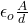where,   A = area = 1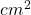=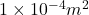d = diameter = 1 mm =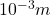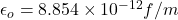Hence, putting the given values into the above formula as follows.

C ==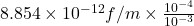=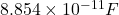Now, we will calculate the energy stored in the capacitor as follows.

U =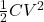=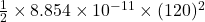=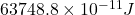or,        =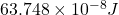Thus, we can conclude that energy stored on each capacitor is.